[BACK]
 Computers, Materials & ContinuaDOI:10.32604/cmc.2022.023837Article

Smart Bubble Sort: A Novel and Dynamic Variant of Bubble Sort Algorithm

College of Computing and Informatics, Saudi Electronic University, Riyadh, Saudi Arabia
*Corresponding Author: Mohammad Khalid Imam Rahmani. Email: m.rahmani@seu.edu.sa
Received: 23 September 2021; Accepted: 02 November 2021

Abstract: In the present era, a very huge volume of data is being stored in online and offline databases. Enterprise houses, research, medical as well as healthcare organizations, and academic institutions store data in databases and their subsequent retrievals are performed for further processing. Finding the required data from a given database within the minimum possible time is one of the key factors in achieving the best possible performance of any computer-based application. If the data is already sorted, finding or searching is comparatively faster. In real-life scenarios, the data collected from different sources may not be in sorted order. Sorting algorithms are required to arrange the data in some order in the least possible time. In this paper, I propose an intelligent approach towards designing a smart variant of the bubble sort algorithm. I call it Smart Bubble sort that exhibits dynamic footprint: The capability of adapting itself from the average-case to the best-case scenario. It is an in-place sorting algorithm and its best-case time complexity is Ω(n). It is linear and better than bubble sort, selection sort, and merge sort. In average-case and worst-case analyses, the complexity estimates are based on its static footprint analyses. Its complexity in worst-case is O(n2) and in average-case is Θ(n2). Smart Bubble sort is capable of adapting itself to the best-case scenario from the average-case scenario at any subsequent stages due to its dynamic and intelligent nature. The Smart Bubble sort outperforms bubble sort, selection sort, and merge sort in the best-case scenario whereas it outperforms bubble sort in the average-case scenario.

Keywords: Sorting algorithms; smart bubble sort; footprint; dynamic footprint; time complexity; asymptotic analysis

1  Introduction

The digital world is producing a huge amount of data from almost every field of life and databases are storing those data in computer-based information systems. As said by Knuth in his book , “virtually every important aspect of programming arises somewhere in the context of sorting or searching”, many improvements have been conducted in sorting and searching algorithms since the advent of programming [2,3]. Sorting various records in different application domains consumes 25% to 50% of the computational resources worldwide as mentioned in [2,4]. It is argued that a single sorting algorithm cannot perform well in every situation that is why for a given scenario, an appropriate sorting algorithm must be applied . For example, bubble sort, insertion sort, and selection sort are best suited to small input data whereas quick sort and merge sort perform better when applied to a large volume of data [2,59]. Researchers have been working to design more efficient algorithms for decades. Currently, new sorting and searching algorithms, as well as efficient modifications, have been proposed by the computer science community. In this connection, Mohammed et al.  developed a Bidirectional Conditional Insertion Sort algorithm, which demonstrated O(n1.5) time complexity in average case scenarios for normal and uniform distributed data. Appiah et al.  have designed a new algorithm: Magnetic Bubble sort with enhancements of bubble sort in the case where redundancies occur in the list. Alotaibi et al.  have proposed a new in-place sorting algorithm, which performs better than bubble sort and selection sort but similar to Insertion sort. Ranaa et al.  have developed a new approach called MinFinder to overcome some of the weaknesses of some conventional algorithms in terms of stability, computational time, and complexity analysis. Cheema et al.  have employed a hybrid approach that takes a minimum number of comparisons with less time and space complexity to sort example data with merge sort and bi-directional bubble sort approaches. Faujdar et al.  have evaluated the existing sorting algorithms using repeated data considering the average-case, worst-case, and best-case scenarios. Sodhi et al.  have designed Enhanced Insertion sort that achieved O(n) worst-case time complexity and O(n1.585) average time complexity showing better performance compared to insertion sort.

In this article, a novel and dynamic variant of bubble sort is being proposed, which I call Smart Bubble sort. In the best-case scenario, our proposed algorithm will iterate only once and will terminate when it detects that the data is already in the sorted order. It has the capability of transforming itself from an average-case scenario to the best-case scenario when it detects that the remaining elements are now sorted after bubbling up some of the elements to their sorted positions during the sorting procedure. I have devised the term dynamic footprint to refer to this concept. The remaining of the paper is structured as follows. In Section 2, preliminary concepts have been discussed. In Section 3, the proposed algorithm is introduced and elaborated along with an example illustration of the working procedure followed by a detailed asymptotic analysis. In Section 4, a description of the new dataset is provided, the experimental setup is explained, and empirical results have been highlighted. In Section 5, conclusions are drawn at the end of the article.

2  Preliminaries

Searching and sorting of database entries are the two direct applications of sorting algorithms. Broader applications of sorting techniques are for the solution of many complex problems in the fields of information retrieval [5,1719], image retrieval , image processing [26,27], database systems, networking , management information systems, decision support systems, operations research and optimization problems . Similarly, sorting algorithms play an important role in educational subjects like design & analysis of algorithms and data structures & programming where the problems require the use of syntax/semantics of every important programming construct related to any programming language. Bubble sort, selection sort, and exchange sort are best suited to data samples of small to medium sizes. These algorithms are comparison-based sorting algorithms; therefore, their lower bound performance is proven to be O(nlogn). A small number of algorithms exist, which claim to have linear bound, however, they are designed for some special cases of input data. Sorting algorithms with O(n) time complexity are considered best performing algorithms. On the other hand, algorithms with O(n2) time complexity are thought to be bad performers whereas algorithms with O(nlogn) time complexity are identified as good algorithms.

2.1 Performance Measurement and Analysis of Sorting Algorithms

The performance of an algorithm is estimated in terms of computational complexity that is measured as its time complexity and space complexity. The time complexity of an algorithm is more important because the availability of sufficiently large memory space in the current era of technology is not a big deal. The efficiency of an algorithm is always expressed as a function of its input size as T(n) or S(n); where n is the input size, T(n) and S(n) are time and space complexities, respectively.

The relative efficacy of sorting algorithms can be characterized by the order of growth of their running times. While calculating the exact running times of a very large volume of input data, the input itself dominates the coefficients and other lower-order terms. For measuring the efficiency of algorithms and comparing the performance of two or more sorting algorithms, the order of growth of their running times is significantly used. However, this technique is not useful when the order of growth of two or more algorithms is the same. On such occasions, the determination of the exact running time of the algorithms is essential which I have done in this article. Asymptotic efficiency of an algorithm refers to the estimation of the order of growth of the running time for very large inputs . That is, I am concerned with how the running time of an algorithm increases with the size of the input in the limit, as the size of the input increases without bound. Usually, an algorithm that is asymptotically more efficient will be the best choice for all but very small inputs. To compare different algorithms for the problem of sorting, I break the analysis of algorithms into three different cases so that I can formulate a way that can provide a better estimate of the resources required. In the first case known as the best-case, the minimum number of computational steps are taken by the algorithm because the input data is sorted in the same order which would be the expected outcome of the algorithm. In the second case known as the average case, the average number of computational steps are taken for the algorithm. This is the most complex kind of analysis that is often based on the probability theory because the input data is randomly collected without any explicit ordering. In the third case known as the worst-case, the maximum number of computational steps are taken by the algorithm. This case is analyzed most often because the maximum time complexity of the algorithm matters the most where the input data is sorted in reverse order.

2.2 Internal Sorting Algorithms

Internal sorting algorithms maintain the entire sequence of data into the main memory of the computer. These algorithms are based on a comparison of items and are further grouped into simple or complex categories. The simple ones are mainly iterative, simple to understand, and easy to implement but their time complexity is more i.e., O(n2). Bubble sort, Selection sort, and Insertion sort are in this category. The complex ones are mainly divide-and-conquer based and difficult to implement but their time complexity is less i.e., O(nlogn). Quicksort, Merge sort, and Heapsort algorithms are in this category.

3  The Proposed Algorithm

In this section, I have first identified the problem of the bubble sort algorithm with its detailed asymptotic analysis. Next, I described our idea about the Smart Bubble sort algorithm and provided its detailed asymptotic analysis with a proper derivation of best case, worst case, and average case notations. A sorting algorithm consists of instructions arranged in a sequence that puts the input data elements in an ordered sequence. Designing an efficient sorting algorithm is necessary for the optimal performance of different computer applications that require sorted data elements to smoothly perform their operations. Sorting algorithms are usually selected based on their computational requirements and ease of implementation. The efficiency of a comparison-based sorting algorithm can be enhanced by reducing either the number of comparison operations or swapping operations or both.

3.1 Problem with Bubble Sort

I start with the formal description of the Bubble sort algorithm in terms of its steps as below:

BUBBLE-SORT(A, n)

A is an array of size n; each element of the array will be sorted after the algorithm gets terminated.

Step 1. for i = 1 to n − 1 do

Step 2.  for j = 1 to n – i − 1 do

Step 3.     if A[j] > A[j+1] then

Step 4.       val = A[j]

Step 5.       A[j] = A[j+1]

Step 6.       A[j+1] = val

It is well known that bubble sort is considered the slowest sorting algorithm because it performs the maximum number of comparisons without looking at the instance of input data. It fails to exploit the instance of input data in the best case and the average case. I have highlighted these phenomena in Figs. 1 and 2 through simple and effective illustrations where the steps of bubble sort are shown on the left side and that of Smart Bubble sort are shown on the right side of the figures.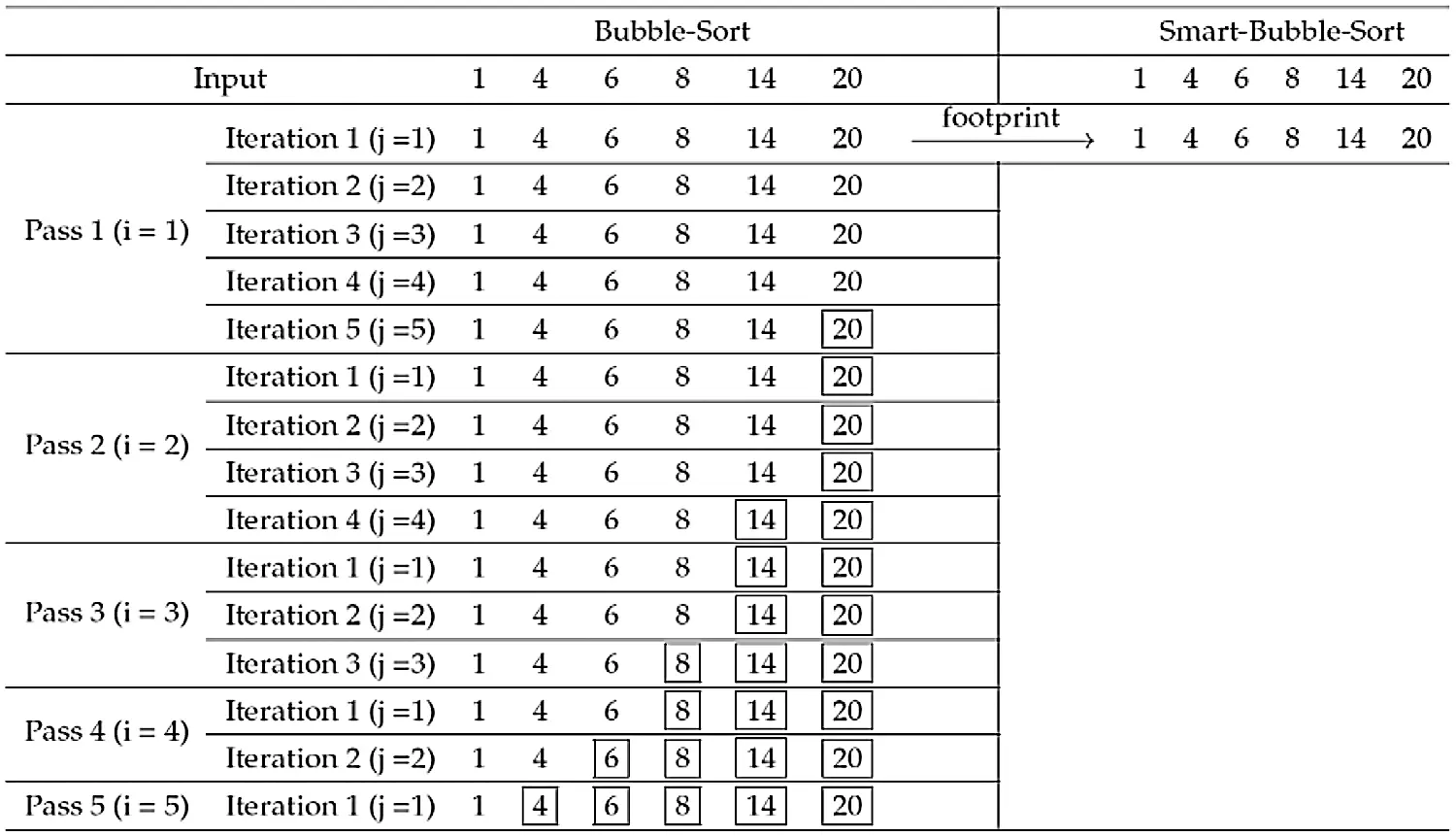Figure 1: Illustration of the footprint of smart-bubble-sort in case of sorted data

In Fig. 1, I can see that the data is already sorted. However, bubble sort will go through all the required passes and steps even if the data is already sorted. On the other hand, Smart Bubble sort will perform only one iteration (Please see Iteration 1 of Pass 1 in Fig. 1) and will not continue once it detects that the data is already sorted. Similarly, the data instance in Fig. 2 is nearly sorted. Again, bubble sort will go through all the passes and steps to complete its operation blindly. After bubbling up the element 20 to the highest position of the array, the remaining elements, 1, 4, 6, 8, and 14 are now in their proper positions of the sorted array at the end of Iteration 2 of Pass 2. Bubble sort is not able to exploit this advantage. It will complete all the remaining iterations and steps blindly doing no further swap operation. However, Smart Bubble sort will exploit this situation to its advantage through its dynamic footprint detection capability and will terminate without performing the remaining iterations and passes.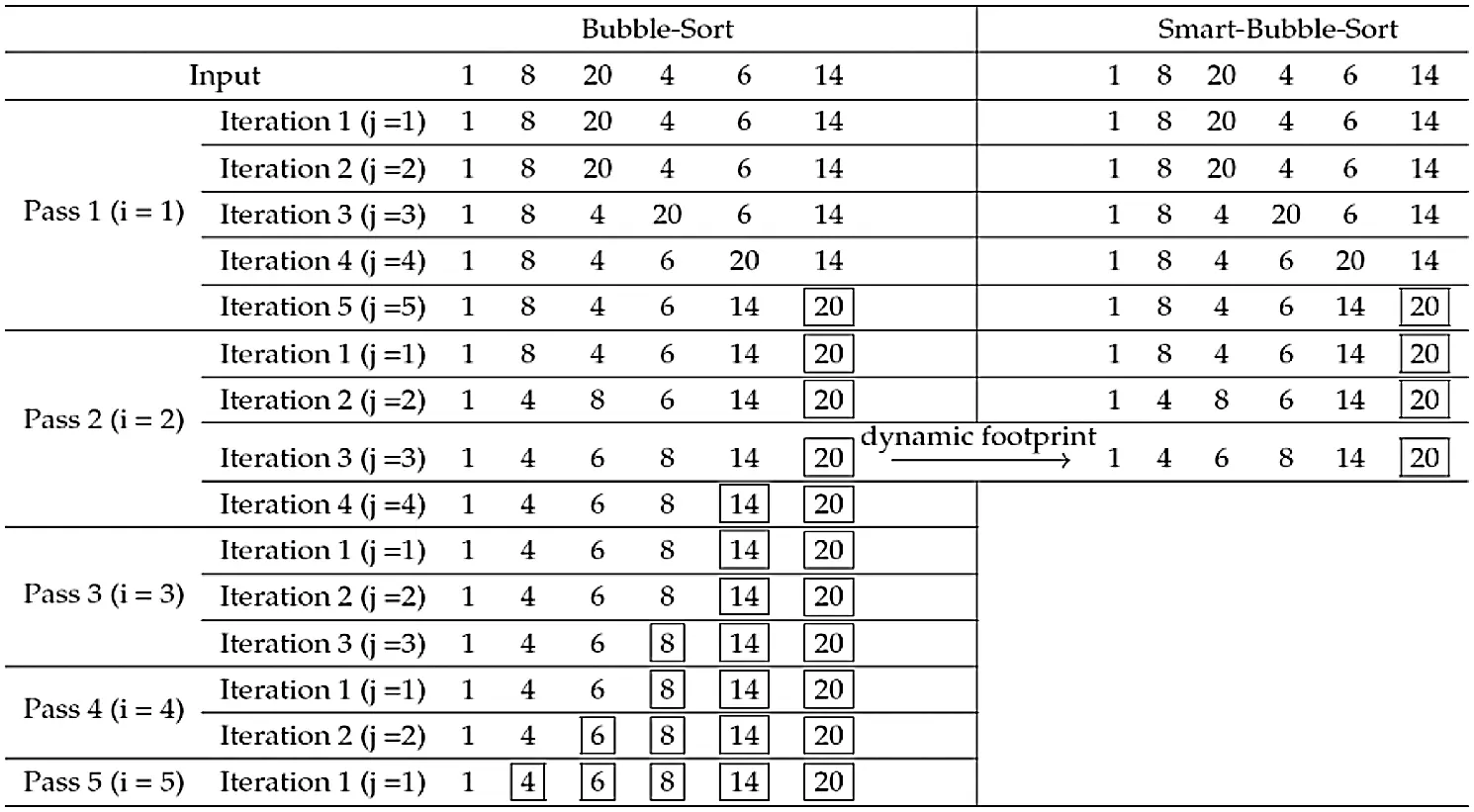Figure 2: Illustration of the dynamic footprint of smart-bubble-sort in case of nearly sorted data

3.2 Analysis of Bubble Sort

Analysis of the algorithms is shown as the general expression of the cost function in terms of the input data size in the form of asymptotic notations Ω(best case), Θ(average case), and O(worst case). The computational complexity for individual steps is provided in Tab. 1 that corresponds to the algorithm provided in BUBBLE-SORT(A, n).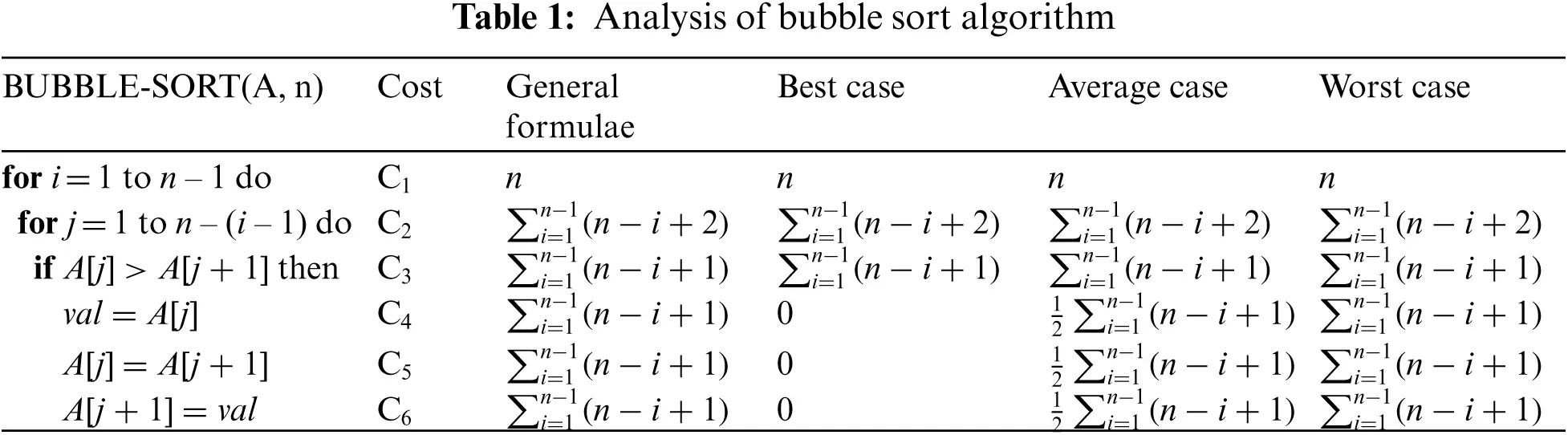3.2.1 Best Case Analysis

The best case of input instance for the sorting algorithms occurs when the elements are sorted in the same order that the algorithm will produce. Therefore, T(n) for the best case can be derived as below:

T(n)=C1n+C2[(n+1)(n+2)23]+C3[n(n+1)21]=C2+C32n2+[C1+3C2+C32]n+(2C2C3)

T(n)=An2+Bn+C)(1)

where, A=C2+C32, B=C1+3C2+C32, C=2C2C3

Since Eq. (1) is a quadratic equation, the time complexity of Bubble sort in best-case is O(n2).

3.2.2 Worst Case Analysis

The worst case of input instance for the sorting algorithms occurs when the elements are sorted in the opposite order that the algorithm will produce. Therefore, T(n) for the worst case can be derived as below:

T(n)=C1n+C2[ (n+1)(n+2)23 ]+C3[ n(n+1)21 ]+C4[ n(n+1)21 ]+C5[ n(n+1)21 ]+C6[ n(n+1)21 ]           =C2+C3+C4+C5+C62n2+[ C1+3C2+C3+C4+C5+C62 ]n+(2C2C3C4C5C6)

T(n)=An2+Bn+C(2)

where, A=C2+C3+C4+C5+C62, B=C1+3C2+C3+C4+C5+C62, C=2C2C3C4C5C6

Eq. (2) is a quadratic equation so its time complexity is O(n2).

3.2.3 Average Case Analysis

The average case of input instance for the sorting algorithms occurs when the elements are arranged in random order. This is the most expected case. Therefore, T(n) for the average case can be derived as below:

T(n)=C1n+C2[ (n+1)(n+2)23 ]+C3[ n(n+1)21 ]+C42[ n(n+1)21 ]+C52[ n(n+1)21 ]+C62[ n(n+1)21 ]           =[ C2+C32+C4+C5+C64 ]n2+[ C1+3C2+C32+C4+C5+C64 ]n+[ 2C2C3C4+C5+C62 ]

T(n)=An2+Bn+C(3)

where, A=C2+C32+C4+C5+C64, B=C1+3C2+C32+C4+C5+C64, C= 2C2C3C4+C5+C62

Since Eq. (3) is a quadratic equation, the algorithm's time complexity in the average case is O(n2).

3.3 The Concept of Smart Bubble Sort

In this section, I have materialized the idea of our proposed Smart Bubble sort algorithm. I begin with the description of the algorithm followed by its asymptotic analysis.

The bubble sort algorithm uses n−1 passes for sorting n numbers. In each pass, the number of iterations to compare the key values are (n-pass number). For controlling the passes of the algorithm, the outer for loop is used. The inner for loop is used for making the actual comparisons for swapping the adjacent key values whenever they are found out of order as shown in BUBBLE-SORT(A, n). Bubble sort is considered the slowest algorithm in average-case and best-case scenarios. But its best-case, as well as average-case running times, can be improved by doing some smart enhancements in the classical bubble sort algorithm. The bubble sort does unnecessary work even if the input data is already sorted as demonstrated in Figs. 1 and 2. It keeps on comparing the items blindly even if the remaining items are already sorted thereby losing the opportunity of exploiting the scenario for terminating it quickly whereas there is no need of running the remaining passes. The Smart Bubble sort algorithm exploits the scenarios and terminates quickly giving much rise in its performance against not only bubble sort but other sorting algorithms as well. In case, the algorithm completes a pass for the remaining elements of the array without detecting any swapping of adjacent elements, it is concluded that the array is sorted and so the algorithm should stop immediately. Similarly, the Smart Bubble sort can transform the average-case into best-case whenever it detects that no swap has been performed at any stage of the algorithm execution as shown in Figs. 1 and 2.

To implement the Smart Bubble sort procedure, I have used a boolean variable flag to detect the swapping of elements. The variable will be set to true at the beginning of every pass and if a swap is detected in that pass, it would be reset to false. If at the end of a pass, the variable is found true, then it would be concluded that no swapping of elements has occurred and hence the algorithm is terminated immediately.

A formal description of the Smart Bubble sort algorithm is given as follows where the order of sorting is considered as ascending order.

Step 1. For n−1 number of passes, carry out each pass.

Step 2. Bubble up the largest element to the top position.

Step 3. If during the bubbling up no swap is encountered, Exit.

Step 4. Else, carry out the next pass.

The formal algorithm for Smart Bubble sort is provided in SMART-BUBBLE-SORT(A, n).

SMART-BUBBLE-SORT(A, n)

A is an array of size n; elements of the array will be sorted in increasing order after the algorithm gets terminated.

Step 1.   for i =1 to n – 1 do

Step 2.      flag = true

Step 3.      for j =1 to ni – 1 do

Step 4.         if A[j] > A[j + 1] then

Step 5.            flag = false

Step 6.            val = A[j]

Step 7.            A[j] = A[j + 1]

Step 8.            A[j + 1] = val

Step 9.      if flag then

Step 10.         break

The time complexity of Smart Bubble sort is Ω(n) in the best case, O(n2) in the worst case, and Θ(n2) in the average case. It is worth noting that the Smart Bubble sort is capable of converting itself from an average-case scenario to the best-case scenario as discussed earlier. This is because the Smart Bubble sort detects the dynamic footprint in its data instance during the execution of the algorithm well before the end of the execution.

3.4 Analysis of Smart Bubble Sort

Analysis of the algorithms is shown as the general expression of the cost function in terms of the input data size in the form of asymptotic notations Ω(best case), Θ(average case), and O(worst case). The computational complexity for individual steps is provided in Tab. 2 that corresponds to the algorithm provided in SMART-BUBBLE-SORT(A, n).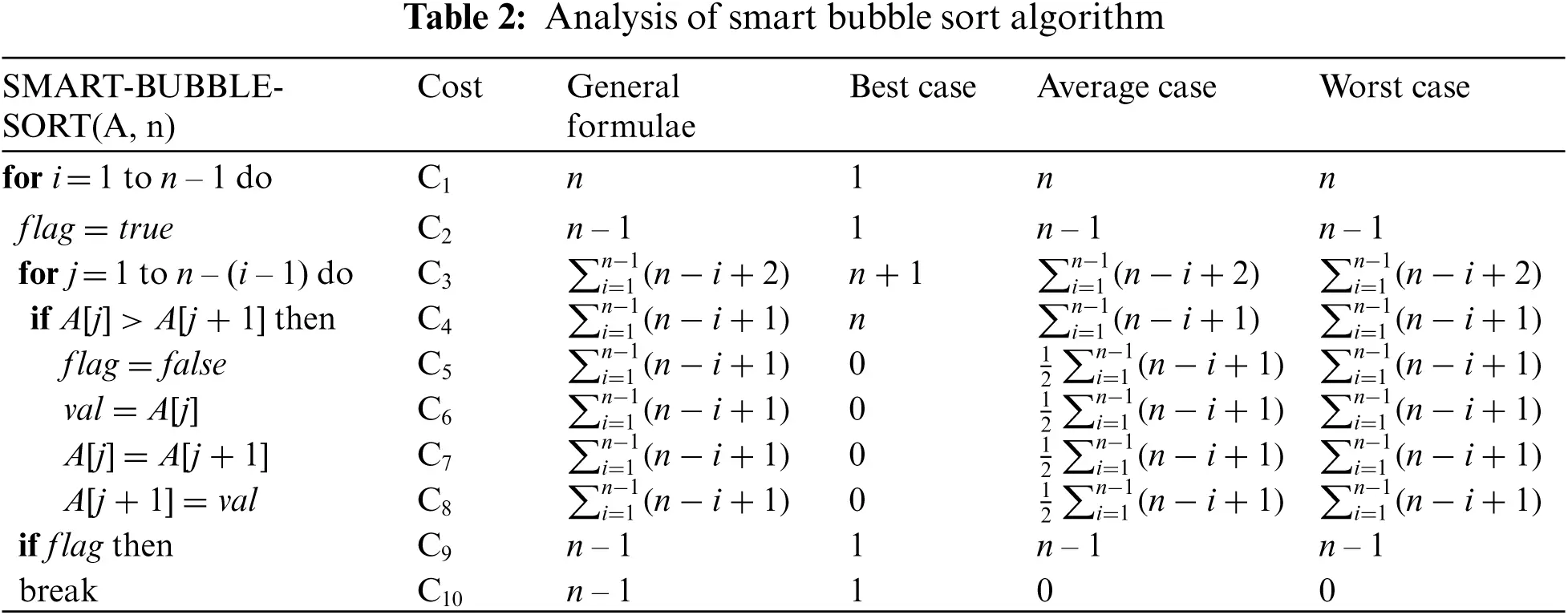3.4.1 Best Case Analysis

The best-case scenario for the algorithm arises when the input array is sorted in the same order in which the output is desired. The algorithm checks this scenario by using a boolean variable flag, which is initialized to true at the beginning of the external for loop (for Passes). Inside the internal for loop (for comparison and swapping) at step 3 when any two adjacent elements are found out-of-order by the if-then structure (i.e., if A[j] > A[j + 1]) of step 4 then these elements are swapped and the flag variable assumes a false value. In case, the value of the variable flag is found true by the if-then structure of step 9, it is concluded that the remaining elements of the array are already sorted. Therefore, the Smart Bubble sort algorithm should stop immediately. This is ensured through the break statement of step 10. As a result, the algorithm does the minimum possible work due to the ability to detect dynamic footprint smartly.

Since the if-then structure of step 4 will never be satisfied in this case, steps 5 through 8 will not be contributing anything to the overall time complexity of the algorithm. Therefore, the total time taken by the algorithm is computed by taking the sum of the products of the corresponding time (cost) and repetition for the contributing steps only. Mathematically, the expression for the time complexity can be derived as follows:

T(n)=C1+C2+C3(n+1)+C4n+C9+C10=(C3+C4)n+(C1+C2+C3+C9+C10)

T(n)=An+B)(4)

where, A=C3+C4, B=C1+C2+C3+C9+C10

Since Eq. (4) is a linear equation, therefore, the coefficient of the highest order term and the constant term are neglected to get the asymptotic notation of the algorithm, which is Ω(n). Therefore, the time complexity of Smart Bubble sort in the best-case is Ω(n).

3.4.2 Worst Case Analysis

The worst case of an input instance for sorting algorithms occurs when the input array is sorted in descending order and the output is desired in ascending order or vice-versa. The algorithm will have to do the maximum work in this case. The for loop of step 1 is tested n times with n − 1 time for executing its body and one more time when the loop condition becomes false. Steps 2 and 9 will be executed n − 1 time. Since each pair of adjacent elements will be out-of-order, the number of swapping will be equal to the number of comparisons. So, the if-then structure of step 9 will never be satisfied and as a result, the break statement of step 10 will never be executed. Steps 3 through 8 will be executed for the maximum possible number of times, which are i=1n1(ni+2) for the step 3 and i=1n1(ni+1) for the rest of the steps. Mathematically, the expression for the time complexity can be derived as follows:

T(n)=C1n+C2(n1)+C3[ (n+1)(n+2)23 ]+C4[ n(n+1)21 ]+C5[ n(n+1)21 ]+C6[ n(n+1)21 ]+C7[ n(n+1)21 ]+C8[ n(n+1)21 ]+C9[ n(n+1)21 ]+C10*0           =C3+C4+C5+C6+C7+C8+C92n2+[ C1+C2+3C3+C4+C5+C6+C7+C8+C92 ]n+(2C3C4C5C6C7C8C9)

T(n)=An2+Bn+C(5)

where, A=C3+C4+C5+C6+C7+C8+C92, B=C1+C2+3C3+C4+C5+C6+C7+C8+C92, and C=2C3C4C5C6C7C8C9.

Since Eq. (5) is a quadratic equation, therefore, the coefficient of the highest order term and the lower order terms are neglected to get the asymptotic notation of the algorithm, which is O(n2). Therefore, the time complexity of Smart Bubble sort in the worst case is O(n2).

3.4.3 Average Case Analysis

The average case of input instance for the sorting algorithms occurs when the elements are arranged in random order. This is the most expected case. The algorithm might have to do as much work as in the worst case . However, I have safely assumed that only half of the adjacent elements are out-of-order. Therefore, the number of swapping will become almost half of the number of comparisons. Similar to the worst-case analysis, the for loop in step 1 is tested n times and steps 2 and 9 will be executed n – 1 time. Step 3 will be executed for i=1n1(ni+2) times and steps 4 through 8 will be executed for i=1n1(ni+1)times. Unlike the worst-case scenario, the if-then structure of step 9 may be satisfied at any successive pass after the first pass. When this happens, the footprint of the algorithm changes to the best-case scenario, and the break statement of step 10 is executed and as a result, the algorithm successfully terminates. This is the situation, which I call the dynamic footprint of the Smart Bubble sort. The dynamic footprint is defined as the ability to convert the footprint from one case of input instance to another case of input instance. Therefore, the total time taken by the algorithm is computed by taking the sum of the products of the corresponding time (cost) and repetition for the contributing steps only. Mathematically, the expression for the time complexity can be derived as given below:

T(n)=C1n+C2(n1)+C3[ (n+1)(n+2)23 ]+C4[ n(n+1)21 ]+C52[ n(n+1)21 ]+C62[ n(n+1)21 ]+C72[ n(n+1)21 ]+C82[ n(n+1)21 ]+C9[ n(n+1)21 ]+C10*0           =[ C3+C4+C92+C5+C6+C7+C84 ]n2+[ C1+C2+3C3+C4+C92+C5+C6+C7+C84 ]n+[ 2C3C4C9+C5C6C7C82 ]

T(n)=An2+Bn+C(6)

where, A=C3+C4+C92+C5+C6+C7+C84,B=C1+C2+3C3+C4+C92+C5+C6+C7+C84, and C=2C3C4C9+C5C6C7C82.

Since Eq. (6) is a quadratic equation, therefore, the coefficient of the highest order term and the lower order terms are neglected to get the asymptotic notation of the algorithm, which is Θ(n2). Therefore, the time complexity of Smart Bubble sort in the average case is Θ(n2).

4  Results and Discussion

In this section, I have constructed a new dataset containing random, sorted, and reverse sorted data as input data to the algorithms. Then I have provided details about the experimental setup. Lastly, I have discussed the results.

4.1 Dataset

Using a perfect dataset is one of the important considerations for establishing an experimental setup for measuring the performance analysis of computer algorithms. In this paper, I have used datasets of randomly created positive integer values generated by a dedicated Java program, which are stored in text format. The datasets are of 3 categories of sizes 500, 2500, 5000, 50000, 100000, 625000, 1250000, and 2500000.

a. Random sets: Sets of the specified sizes were created in random order to analyze the average case scenario.

b. Sorted sets: The Random sets were first sorted and then used for the analysis of the best-case scenario.

c. Reverse Sorted sets: The Sorted sets were reversed and then used for the analysis of the worst-case scenario.

I have designed a Java program that used the Random class along with its nextInt() method. This class was instantiated for calling the nextInt() method. The value of the argument range in the nextInt() method was given as 2147483647 to maintain as much uniqueness of elements in the data set as possible.

Besides, I have created another similar dataset with approximately 50% sorted data items to provide evidence of dynamic footprint detection capability for which the results are provided in Section 4.2.4.

4.2 Experimental Setup

The proposed algorithm's steps are written in a formal way suitable to be implemented in any programming language but its Java implementation is the most efficient one in terms of CPU time (measured in nanoseconds) and memory requirement [32,33]. I have used IntelliJ IDEA version 11.0.9 with JDK 1.8.0_231 for implementing the algorithms and testing & validating the results. In our implementation, the variable, flag of type boolean is used to check if any swapping is made inside the inner for loop or not. A boolean variable may assume only two values; true or false. So, its implementation requires just 1 bit of memory  whereas C/C++ implementation  will use either short or int, which requires 2 to 4 bytes of memory.

The experiments are performed on a 3.6 GHz Intel Core i9 (9th generation) processor with 64 GB RAM, and Windows 10 (64-bit OS) platform.

4.2.1 Performance Analysis of Smart Bubble Sort on Sorted Data

The experimental results for best-case analysis on sorted data are presented in Supplementary Tab. 1 where the names of the sorting algorithms are given along the top row and the data sizes are arranged along the first column. The best-performing values are highlighted in blue. As evident from the obtained results, Smart Bubble sort is outperforming all the listed algorithms by a huge margin in terms of the number of comparisons, swapping, and CPU clock time. It can be noted down that from this point onward swapping will refer to merging operation in case of merge sort analysis. The growth of the Smart Bubble sort algorithm is linear to the size of the input data in the best-case scenario. Therefore, CPU clock time is the least. This is the edge of the Smart Bubble sort over bubble sort and the rest of the algorithms under consideration. This is because the number of swapping in Bubble sort is zero but the number of comparisons is higher than the Smart Bubble sort. In the case of merge sort and selection sort, both the number of comparisons and swapping are higher than those of the Smart Bubble sort. For a comprehensive understanding of better performing results and achievements of the Smart Bubble sort, I illustrated the number of comparisons, swapping, and CPU clock time for all data sizes including 500, 2500, 5000, 50000, 100000, 625000, 1250000, and 2500000 in Fig. 3.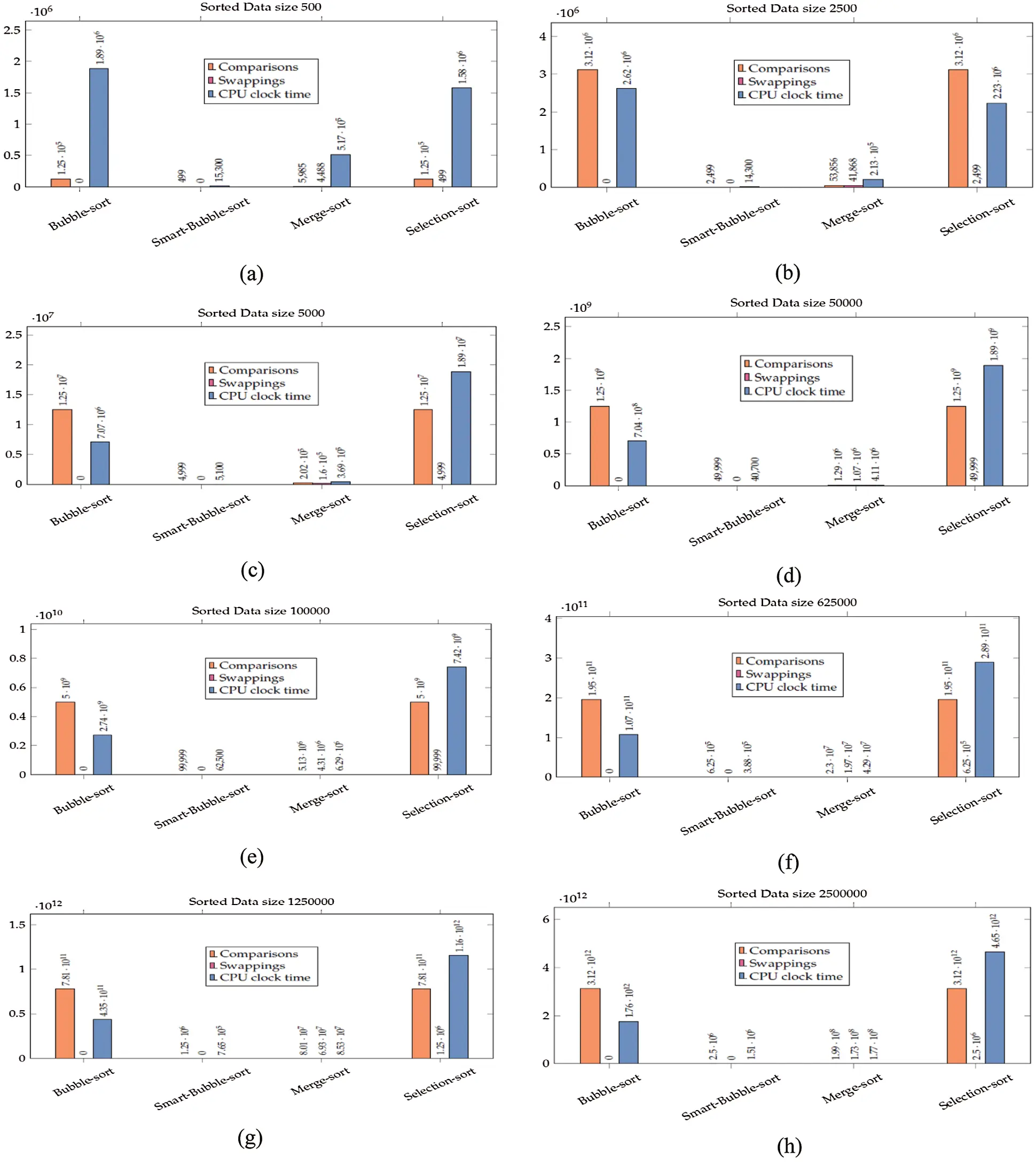Figure 3: Results for applying sorting algorithms on the sorted dataset: (a) data size 500, (b) data size 2500, (c) data size 5000, (d) data size 50000, (e) data size 1000000, (f) data size 625000, (g) data size 150000 and (h) 2500000

4.2.2 Performance Analysis of Smart Bubble Sort on Reverse Sorted Data

The experimental results for worst-case analysis on reverse sorted data are presented in Supplementary Tab. 2 where the names of the sorting algorithms are given along the top row and the data sizes are arranged along the first column. The best-performing values are highlighted in blue. It is evident from the results that in the worst-case scenario, the performance of Smart Bubble sort is much better than bubble sort and selection sort in terms of CPU clock time however, it is much slower than the merge sort algorithm. For data size 500, the CPU clock time is higher for Smart Bubble sort because the number of steps of SMART-BUBBLE-SORT(A, n) is more than those of BUBBLE-SORT(A, n). Therefore, the cost of the coefficient is higher for Smart Bubble sort as evident from Eqs. (2) and (5). However, for the data sizes greater than 500, this overhead is overtaken by the performance of the algorithm as evident from Supplementary Tab. 2. The best values of CPU clock time for Smart Bubble sort are given in blue whereas the value where the CPU clock time is more for Smart Bubble sort while processing data size 500 is given in red. For a comprehensive understanding of the achieved results, I highlighted the number of comparisons, swapping, and CPU clock time for all sizes of reverse sorted data including 500, 2500, 5000, 50000, 100000, 625000, 1250000, and 2500000 in Fig. 4.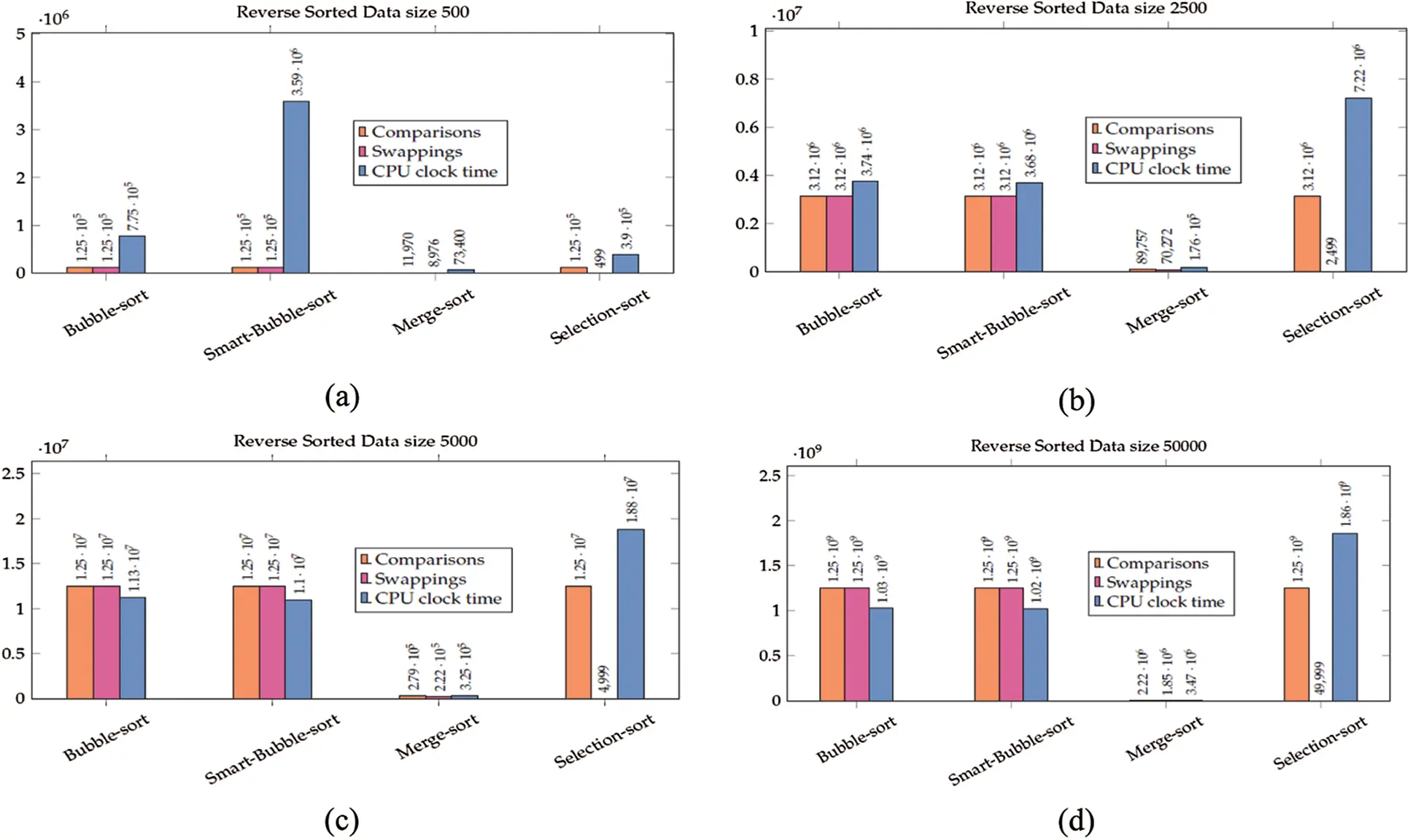Figure 4: Results for applying sorting algorithms on the reverse sorted dataset: (a) data size 500, (b) data size 2500, (c) data size 5000, (d) data size 50000, (e) data size 1000000, (f) data size 625000, (g) data size 150000 and (h) 2500000

4.2.3 Performance Analysis of Smart Bubble Sort on Random Data

The experimental results for average-case analysis on random data are demonstrated in Supplementary Tab. 3 where the names of the sorting algorithms are mentioned along the top row whereas the data sizes are presented along the first column. The best-performing values are highlighted in blue. The results show the superior performance of Smart Bubble sort in terms of the number of comparisons and CPU clock time over bubble sort. It can be observed from Supplementary Tab. 3 that the Smart Bubble sort is performing a lesser number of comparisons than the Bubble sort as well as Selection sort for all data sizes. However, CPU clock time is lesser for data sizes 50000 and above as compared to the Bubble sort algorithm. In the case of Selection sort and Merge sort, both the number of comparisons and swapping (merging in case of merge sort) are less than those of the Smart Bubble sort. For data size 500, 2500, and 5000, the CPU clock time is higher for Smart Bubble sort despite a lesser number of comparisons. This is because the number of steps of SMART-BUBBLE-SORT(A, n) is more than those of BUBBLE-SORT(A, n). Therefore, the cost of the coefficient is higher for Smart Bubble sort as evident from Eqs. (3) and (6). However, for the data sizes greater than 50000, this overhead is overtaken by the performance of the algorithm as evident from Supplementary Tab. 3. The best values of comparisons and CPU clock time for Smart Bubble sort are given in blue whereas the values where the CPU clock time is more for Smart Bubble sort while processing data size of 500, 2500, and 5000 are given in red. I highlighted the number of comparisons, swapping, and CPU clock time for all sizes of random data including 500, 2500, 5000, 50000, 100000, 625000, 1250000, and 2500000 in Fig. 5.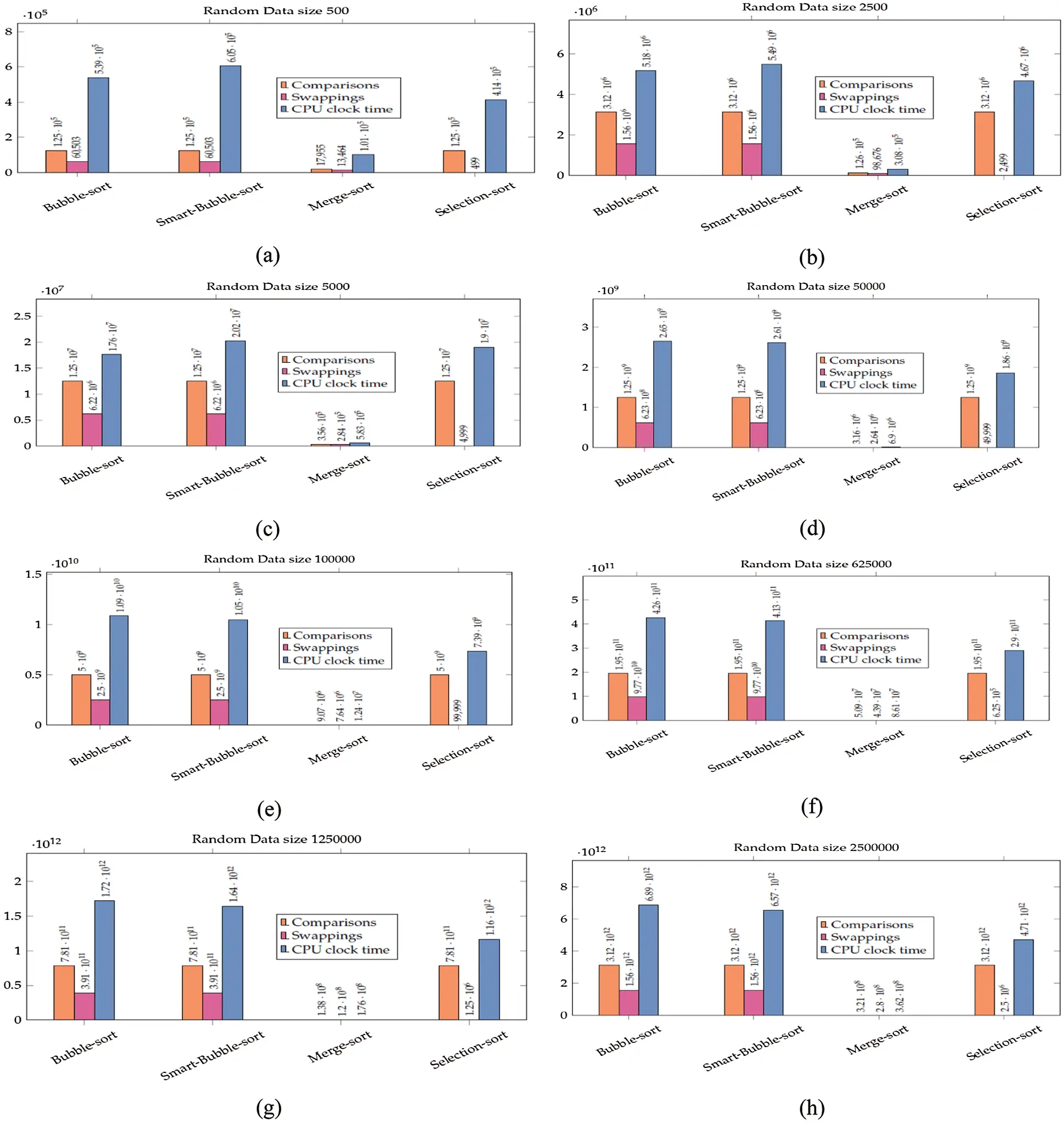Figure 5: Results for applying sorting algorithms on the random dataset: (a) data size 500, (b) data size 2500, (c) data size 5000, (d) data size 50000, (e) data size 1000000, (f) data size 625000, (g) data size 150000 and (h) 2500000

4.2.4 Performance Analysis of Smart Bubble Sort for Validation of Dynamic Footprint

The experimental results for dynamic footprint analysis on partially sorted data are presented in Supplementary Tab. 4 where names of the sorting algorithms are mentioned along the top row and the data sizes are given along the first column. The best-performing values are highlighted in blue. The number of swapping performed by Smart Bubble sort have now become half of the total number of comparisons for a given data as claimed in the column Average Case of Tab. 2 and supported by the results in Supplementary Tab. 4. It is because the input data is now partially sorted to establish a basis for detecting the dynamic footprint by Smart Bubble sort for transforming itself from the average case scenario to the best-case scenario. The number of comparisons performed by Smart Bubble sort is less than those of bubble sort and selection sort algorithms. I can observe that the number of swapping performed by Smart Bubble sort is equal to those of bubble sort. As I discussed previously the Smart Bubble sort can detect the dynamic footprint of the data instance and as a result, it can transform itself from an average case to the best-case scenario. The number of comparisons is less than Bubble sort is because Smart Bubble sort detected that the remaining data items are already sorted so it terminated earlier whereas bubble sort kept on comparing the already sorted elements blindly, which caused more number of comparisons. On the other hand, for data size 500, the CPU clock time is higher for Smart Bubble sort despite a lesser number of comparisons. This is because the number of steps of SMART-BUBBLE-SORT(A, n) is more than those of BUBBLE-SORT(A, n). Therefore, the cost of the coefficient is higher for the Smart Bubble sort. However, for the data sizes greater than 500, this overhead is overtaken by the performance of the algorithm as evident from Supplementary Tab. 4. The best values of comparisons for Smart Bubble sort are given in blue whereas the value where the CPU clock time is more for Smart Bubble sort while processing data size 500 is given in red. It is now evident that the lesser number of comparisons by Smart Bubble sort is due to its dynamic footprint detection capability (Please, refer to Fig. 6).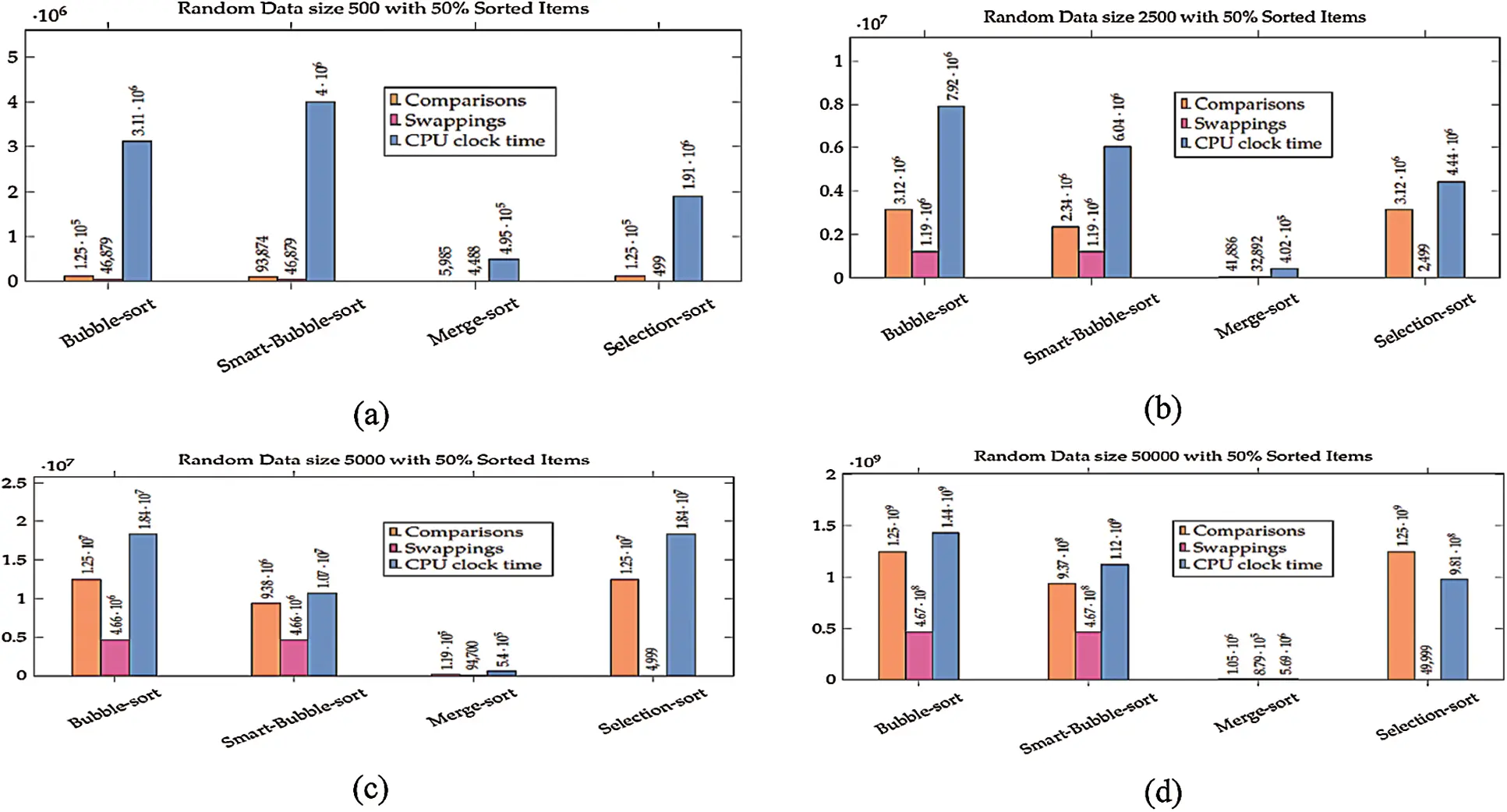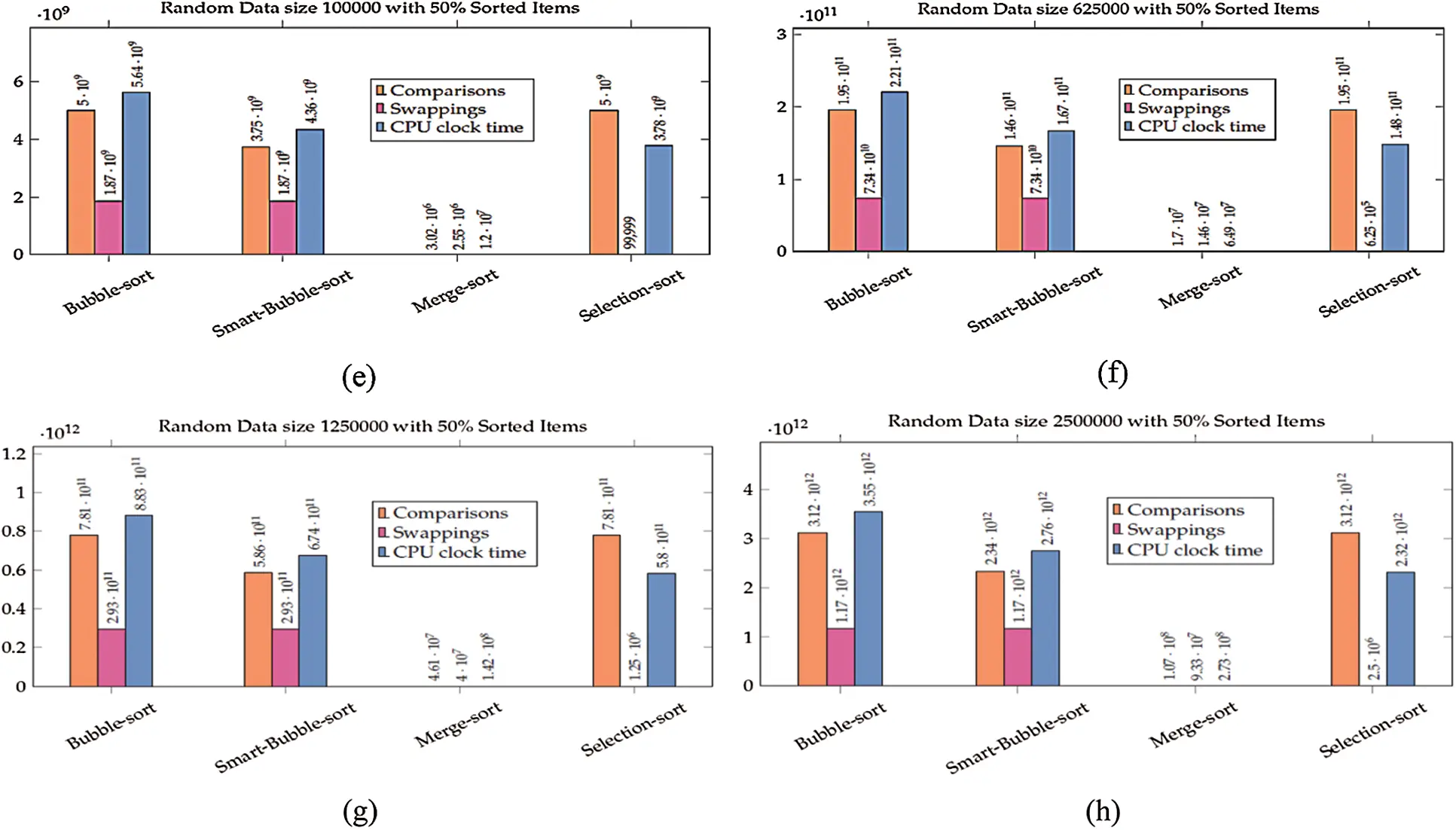Figure 6: Results for applying sorting algorithms on the random dataset with 50% sorted data: (a) data size 500, (b) data size 2500, (c) data size 5000, (d) data size 50000, (e) data size 1000000, (f) data size 625000, (g) data size 150000 and (h) 2500000

5  Conclusion

In this article, I have designed and verified a novel and dynamic variant of the bubble sort algorithm with performance improvements in the best case and average case scenarios while keeping the worst-case performance well within the upper bound. The proposed Smart Bubble sort algorithm demonstrated better performance compared to bubble sort, selection sort, and merge sort in the best case scenario with a time complexity of Ω(n).

To achieve the improvements for the average-case scenario, the concept of dynamic footprint has been elaborated and empirically verified. It is observed that the performance of an algorithm can be enhanced by identifying useful patterns present in the data and consequently exploiting the scenarios for improving the performance and efficiency of the algorithm. Smart Bubble sort can detect the useful patterns in average case data favoring its transformation to the best-case scenario and finally, it can adapt from average case to the best-case scenario. This enables it to terminate early in the sorting process while giving about a 25% performance raise over bubble sort.

Data Availability Statement: The constructed datasets are available at https://drive.google.com/file/d/112pWWW_cDWgM7O3oa80Tn6O1ASR38mEh/view?usp=sharing.

Funding Statement: The author received no specific funding for this study.

Conflicts of Interest: The author declares that he has no conflicts of interest to report regarding the present study.

## References

1. D. E. Knuth, The Art of Computer Programming: Sorting and Searching, vol. 3, Second ed., Boston: Addison-Wesley Professional, 1998.
2. S. S. Skiena, The Algorithm Design Manual, Second ed., London: Springer International Publishing, 2020.
3. D. E. Knuth, The Art of Computer Programming, vol. 4, satisfiability: Fascicle 6, Boston: Addison-Wesley Professional, 2015.
4. R. Sedgewick and K. Wayne, Algorithms: 24-Part Lecture Series, Fourth ed., Boston: Addison-Wesley Professional, 2015.
5. E. B. Koffman and P. A. T. Wolfgang, Data Structures: Abstraction and Design using Java, Fourth ed., Hoboken, New Jersey: Wiley, 2021.
6.    H. Knebl, Algorithms and Data Structures: Foundations and Probabilistic Method for Design and Analysis, First ed., London: Springer International Publishing, 2020.
7. T. H. Cormen, C. E. Leiserson, R. L. Rivest and C. Stein, Introduction to Algorithms, Second ed., Cambridge, USA: MIT Press, 2009.
8.    D. R. Chawdhuri, Java 9 Data Structures and Algorithms, First ed., Birmingham, UK: Packt, 2017.
9. M. T. Goodrich, R. Tamassia and M. H. Goldwasser, Data Structures and Algorithms in Java, Hoboken, New Jersey: John Wiley & Sons, 2014.
10. A. S. Mohammed, S. E. Amrahov and F. V. Celibi, “Bidirectional conditional insertion sort algorithm: An efficient progress on the classical insertion sort,” Future Generation Computer Systems, vol. 71, pp. 102–112, 2017.
11. O. Appiah and E. M. Martey, “Magnetic bubble sort algorithm,” International Journal of Computer Applications, vol. 122, pp. 24–28, 2015.
12. A. Alotaibi, A. Almutairi and H. Kurdi, “Onebyone (OBOA fast sorting algorithm,” Procedia Computer Science, vol. 175, pp. 270–277, 2020.
13. M. S. Ranaa, M. A. Hossinb, S. H. Mahmudc, H. Jahane, A. M. Z. Satterf et al., “Minfinder: A new approach in sorting algorithm,” Procedia Computer Science, vol. 154, pp. 130–136, 2019.
14. S. M. Cheema, N. Sarwar and F. Yousaf, “Contrastive analysis of bubble & merge sort proposing hybrid approach,” in Sixth Int. Conf. on Innovative Computing Technology (INTECH), Dublin, Ireland, IEEE, 2016, pp. 371–375, 2016.
15. N. Faujdar and S. P. Ghrera, “Analysis and testing of sorting algorithms on a standard dataset,” in Fifth Int. Conf. on Communication Systems and Network Technologies, Gwalior, India, IEEE, pp. 962–967, 20
16. T. S. Sodhi, S. Kaur and S. Kaur, “Enhanced insertion sort algorithm,” International Journal of Computer Applications, vol. 64, pp. 35–39, 2013.
17. D. Maxwell, L. Azzopardi and Y. Moshfeghi, “The impact of result diversification on search behaviour and performance,” Information Retrieval Journal, vol. 22, pp. 422–446, 2019.
18. E. Amigó, F. Giner, J. Gonzalo and F. Verdejo, “On the foundations of similarity in information access,” Information Retrieval Journal, vol. 23, pp. 216–254, 2020.
19. H. Tufail, F. Azam, M. W. Anwar, M. N. Zafar, A. W. Muzaffar et al., “A model-driven alarms framework (MAF) with mobile clients support for wide-ranging industrial control systems,” IEEE Access, vol. 8, pp. 174279–174304, 2020.
20. M. K. I. Rahmani, N. Pal and K. Arora, “Clustering of image data using K-means and fuzzy K-means,” International Journal of Advanced Computer Science and Applications, vol. 5, pp. 160–163, 2014.
21. B. H. Yuan and G. H. Liu, “Image retrieval based on gradient-structures histogram,” Neural Computing and Applications, vol. 32, pp. 11717–11727, pp. 1–11, 2020.
22. M. K. I. Rahmani, M. Ansari and A. K. Goel, “An efficient indexing algorithm for cbir,” in IEEE Int. Conf. on Computational Intelligence & Communication Technology, Ghaziabad, India, IEEE, pp. 73–77, 2015.
23. P. Chhabra, N. K. Garg and M. Kumar, “Content-based image retrieval system using ORB and SIFT features,” Neural Computing and Applications, vol. 32, pp. 2725–2733, 2020.
24. M. K. I. Rahmani and M. A. Ansari, “A color based fuzzy algorithm for cbir,” in Confluence 2013: The Next Generation Information Technology Summit (4th International Conference), pp. 363–370, 2013.
25. M. K. I. Rahmani, K. Arora and N. Pal, “A crypto-steganography: A survey,” International Journal of Advanced Computer Science and Application, vol. 5, pp. 149–154, 2014.
26. M. Tahir and A. Idris, “Md-lbp: An efficient computational model for protein subcellular localization from hela cell lines using svm,” Current Bioinformatics, vol. 15, no. 3, pp. 204–211, 2020.
27. M. Tahir, B. Jan, M. Hayat, S. U. Shah and M. Amin, “Efficient computational model for classification of protein localization images using extended threshold adjacency statistics and support vector machines,” Computer Methods and Programs in Biomedicine, vol. 157, pp. 205–215, 2018.
28. F. Samea, F. Azam, M. Rashid, M. W. Anwar, W. H. Butt et al., “A model-driven framework for data-driven applications in serverless cloud computing,” Plos One, vol. 15, no. 8, pp. e0237317, 2020.
29. A. H. Alenezy, M. T. Ismail, S. A. Wadi, M. Tahir, N. N. Hamadneh et al., “Forecasting stock market volatility using hybrid of adaptive network of fuzzy inference system and wavelet functions,” Journal of Mathematics, vol. 2021, pp. 1–10, 2021.
30. K. A. Rashedi, M. T. Ismail, N. N. Hamadneh, S. Wadi, J. J. Jaber et al., “Application of radial basis function neural network coupling particle swarm optimization algorithm to classification of Saudi Arabia stock returns,” Journal of Mathematics, vol. 2021, pp. 1–8, 2021.
31. I. Alhadid, H. Tarawneh, K. Kaabneh, R. Masa'deh, N. N. Hamadneh et al., “Optimizing service composition (sc) using smart multistage forward search (smfs),” Intelligent Automation and Soft Computing, vol. 28, no. 2, pp. 321–336, 2021.
32. V. Sarcar, “Important features in Java's enhancement path,” in Interactive Object-Oriented Programming in Java, London: Springer, pp. 423–439, 2020.
33. A. L. Davis, Modern Programming Made Easy: Using Java, Scala, Groovy, and Javascript, Second ed., New York: Apress, 2020.
34. C. S. Horstmann, Big Java: Early Objects, Seventh ed., Hoboken, New Jersey: John Wiley & Sons, 2018.
35. D. A. CRIS, G. F. Simion and P. E. Moraru, “Run-time analysis for sorting algorithms,” Journal of Information Systems and Operations Management, vol. 9, pp. 1–10, 2015.This work is licensed under a Creative Commons Attribution 4.0 International License, which permits unrestricted use, distribution, and reproduction in any medium, provided the original work is properly cited.